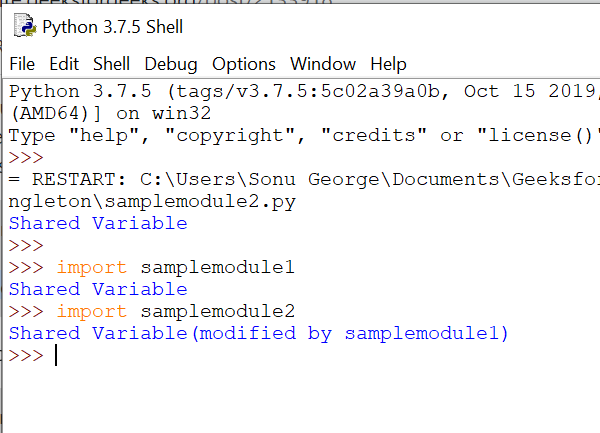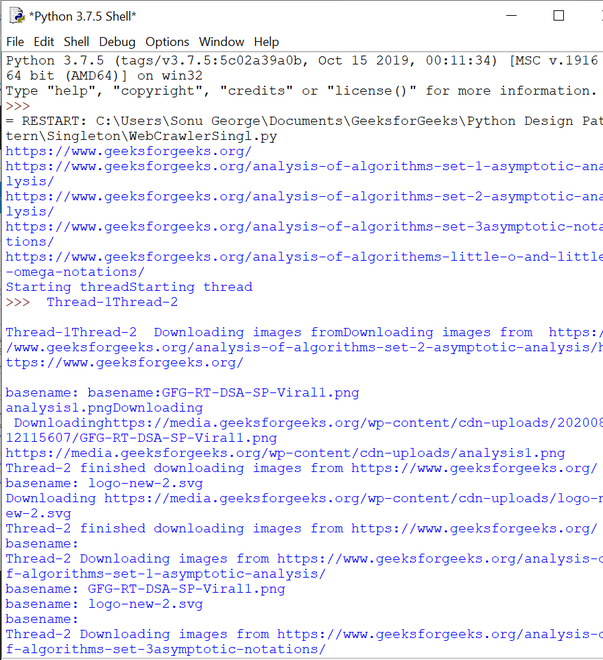Related Articles

# Singleton Pattern in Python – A Complete Guide

• Last Updated : 27 Aug, 2021

A Singleton pattern in python is a design pattern that allows you to create just one instance of a class, throughout the lifetime of a program. Using a singleton pattern has many benefits. A few of them are:

• To create a global point of access for a resource.
• To create just one instance of a class, throughout the lifetime of a program.

Different ways to implement a Singleton:

A singleton pattern can be implemented in three different ways. They are as follows:

• Module-level Singleton
• Classic Singleton
• Borg Singleton

Module-level Singleton:

All modules are singleton, by definition.  Let’s create a simple module-level singleton where the data is shared among other modules. Here we will create three python files – singleton.py, sample_module1.py, and sample_module2.py – in which the other sample modules share a variable from singleton.py.

```## singleton.py
shared_variable = "Shared Variable"```

singleton.py

```## samplemodule1.py
import singleton
print(singleton.shared_variable)
singleton.shared_variable += "(modified by samplemodule1)"```

samplemodule1.py

```##samplemodule2.py
import singleton
print(singleton.shared_variable)```

samplemodule2.py

Let’s look into the output.Here, the value changed by samplemodule1 is also reflected in samplemodule2.

Classic Singleton:

Classic Singleton creates an instance only if there is no instance created so far; otherwise, it will return the instance that is already created. Let’s take a look at the below code.

## Python3

 `class` `SingletonClass(``object``):``  ``def` `__new__(``cls``):``    ``if` `not` `hasattr``(``cls``, ``'instance'``):``      ``cls``.instance ``=` `super``(SingletonClass, ``cls``).__new__(``cls``)``    ``return` `cls``.instance``  ` `singleton ``=` `SingletonClass()``new_singleton ``=` `SingletonClass()` `print``(singleton ``is` `new_singleton)` `singleton.singl_variable ``=` `"Singleton Variable"``print``(new_singleton.singl_variable)`
Output
```True
Singleton Variable```

Here, in the __new__ method, we will check whether an instance is created or not. If created, it will return the instance; otherwise, it will create a new instance. You can notice that singleton and new_singleton return the same instance and have the same variable.

Let’s check what happens when we subclass a singleton class.

## Python3

 `class` `SingletonClass(``object``):``  ``def` `__new__(``cls``):``    ``if` `not` `hasattr``(``cls``, ``'instance'``):``      ``cls``.instance ``=` `super``(SingletonClass, ``cls``).__new__(``cls``)``    ``return` `cls``.instance``  ` `class` `SingletonChild(SingletonClass):``    ``pass``  ` `singleton ``=` `SingletonClass() ``child ``=` `SingletonChild()``print``(child ``is` `singleton)` `singleton.singl_variable ``=` `"Singleton Variable"``print``(child.singl_variable)`

Output
```True
Singleton Variable```

Here, you can see that SingletonChild has the same instance of SingletonClass and also shares the same state. But there are scenarios, where we need a different instance, but should share the same state. This state sharing can be achieved using Borg singleton.

Borg Singleton:

Borg singleton is a design pattern in Python that allows state sharing for different instances. Let’s look into the following code.

## Python3

 `class` `BorgSingleton(``object``):``  ``_shared_borg_state ``=` `{}``  ` `  ``def` `__new__(``cls``, ``*``args, ``*``*``kwargs):``    ``obj ``=` `super``(BorgSingleton, ``cls``).__new__(``cls``, ``*``args, ``*``*``kwargs)``    ``obj.__dict__ ``=` `cls``._shared_borg_state``    ``return` `obj``  ` `borg ``=` `BorgSingleton()``borg.shared_variable ``=` `"Shared Variable"` `class` `ChildBorg(BorgSingleton):``  ``pass` `childBorg ``=` `ChildBorg()``print``(childBorg ``is` `borg)``print``(childBorg.shared_variable)`
Output
```False
Shared Variable```

Along with the new instance creation process, a shared state is also defined in the __new__ method. Here the shared state is retained using the shared_borg_state attribute and it is stored in the __dict__ dictionary of each instance.

If you want a different state, then you can reset the  shared_borg_state attribute. Let’s see how to reset a shared state.

## Python3

 `class` `BorgSingleton(``object``):``  ``_shared_borg_state ``=` `{}``  ` `  ``def` `__new__(``cls``, ``*``args, ``*``*``kwargs):``    ``obj ``=` `super``(BorgSingleton, ``cls``).__new__(``cls``, ``*``args, ``*``*``kwargs)``    ``obj.__dict__ ``=` `cls``._shared_borg_state``    ``return` `obj``  ` `borg ``=` `BorgSingleton()``borg.shared_variable ``=` `"Shared Variable"` `class` `NewChildBorg(BorgSingleton):``    ``_shared_borg_state ``=` `{}` `newChildBorg ``=` `NewChildBorg()``print``(newChildBorg.shared_variable)`

Here, we have reset the shared state and tried to access the shared_variable. Let’s see the error.

```Traceback (most recent call last):
File "/home/329d68500c5916767fbaf351710ebb13.py", line 16, in <module>
print(newChildBorg.shared_variable)
AttributeError: 'NewChildBorg' object has no attribute 'shared_variable'```

Use cases of a Singleton:

Let’s list a few of the use cases of a singleton class. They are as follows:

• Managing a database connection
• File Manager
• Print spooler

Create a Web Crawler using Classic Singleton:

Let’s create a webcrawler that uses the benefit of a classic singleton. In this practical example, the crawler scans a webpage, fetch the links associated with the same website, and download all the images in it. Here, we have two main classes and two main functions.

• CrawlerSingleton: This class acts a classic singleton
• navigate_site: This function crawls the website and fetches the links that belong to the same website. And, finally, it arranges the link to download images.

Apart from the above classes and functions, we use two sets of libraries to parse the web page – BeautifulSoap and HTTP Client.

Have a look at the below code.

Note: Execute the code in your local machine

## Python3

 `import` `httplib2``import` `os``import` `re``import` `threading``import` `urllib``import` `urllib.request``from` `urllib.parse ``import` `urlparse, urljoin``from` `bs4 ``import` `BeautifulSoup` `class` `CrawlerSingleton(``object``):``    ``def` `__new__(``cls``):``        ``""" creates a singleton object, if it is not created,``        ``or else returns the previous singleton object"""``        ``if` `not` `hasattr``(``cls``, ``'instance'``):``            ``cls``.instance ``=` `super``(CrawlerSingleton, ``cls``).__new__(``cls``)``        ``return` `cls``.instance` `def` `navigate_site(max_links ``=` `5``):``    ``""" navigate the website using BFS algorithm, find links and``        ``arrange them for downloading images """` `    ``# singleton instance``    ``parser_crawlersingleton ``=` `CrawlerSingleton()``    ` `    ``# During the initial stage, url_queue has the main_url.``    ``# Upon parsing the main_url page, new links that belong to the``    ``# same website is added to the url_queue until``    ``# it equals to max _links.``    ``while` `parser_crawlersingleton.url_queue:` `        ``# checks whether it reached the max. link``        ``if` `len``(parser_crawlersingleton.visited_url) ``=``=` `max_links:``            ``return` `        ``# pop the url from the queue``        ``url ``=` `parser_crawlersingleton.url_queue.pop()` `        ``# connect to the web page``        ``http ``=` `httplib2.Http()``        ``try``:``            ``status, response ``=` `http.request(url)``        ``except` `Exception:``            ``continue``        ` `        ``# add the link to download the images``        ``parser_crawlersingleton.visited_url.add(url)``        ``print``(url)` `        ``# crawl the web page and fetch the links within``        ``# the main page``        ``bs ``=` `BeautifulSoup(response, ``"html.parser"``)` `        ``for` `link ``in` `BeautifulSoup.findAll(bs, ``'a'``):``            ``link_url ``=` `link.get(``'href'``)``            ``if` `not` `link_url:``                ``continue` `            ``# parse the fetched link``            ``parsed ``=` `urlparse(link_url)``            ` `            ``# skip the link, if it leads to an external page``            ``if` `parsed.netloc ``and` `parsed.netloc !``=` `parsed_url.netloc:``                ``continue` `            ``scheme ``=` `parsed_url.scheme``            ``netloc ``=` `parsed.netloc ``or` `parsed_url.netloc``            ``path ``=` `parsed.path``            ` `            ``# construct a full url``            ``link_url ``=` `scheme ``+``'://'` `+``netloc ``+` `path` `            ` `            ``# skip, if the link is already added``            ``if` `link_url ``in` `parser_crawlersingleton.visited_url:``                ``continue``            ` `            ``# Add the new link fetched,``            ``# so that the while loop continues with next iteration.``            ``parser_crawlersingleton.url_queue ``=` `[link_url] ``+``\``                                                ``parser_crawlersingleton.url_queue``            ` `class` `ParallelDownloader(threading.Thread):``    ``""" Download the images parallelly """``    ``def` `__init__(``self``, thread_id, name, counter):``        ``threading.Thread.__init__(``self``)``        ``self``.name ``=` `name` `    ``def` `run(``self``):``        ``print``(``'Starting thread'``, ``self``.name)``        ``# function to download the images``        ``download_images(``self``.name)``        ``print``(``'Finished thread'``, ``self``.name)``            ` `def` `download_images(thread_name):``    ``# singleton instance``    ``singleton ``=` `CrawlerSingleton()``    ``# visited_url has a set of URLs.``    ``# Here we will fetch each URL and``    ``# download the images in it.``    ``while` `singleton.visited_url:``        ``# pop the url to download the images``        ``url ``=` `singleton.visited_url.pop()` `        ``http ``=` `httplib2.Http()``        ``print``(thread_name, ``'Downloading images from'``, url)` `        ``try``:``            ``status, response ``=` `http.request(url)``        ``except` `Exception:``            ``continue` `        ``# parse the web page to find all images``        ``bs ``=` `BeautifulSoup(response, ``"html.parser"``)` `        ``# Find all tags``        ``images ``=` `BeautifulSoup.findAll(bs, ``'img'``)` `        ``for` `image ``in` `images:``            ``src ``=` `image.get(``'src'``)``            ``src ``=` `urljoin(url, src)` `            ``basename ``=` `os.path.basename(src)``            ``print``(``'basename:'``, basename)` `            ``if` `basename !``=` `'':``                ``if` `src ``not` `in` `singleton.image_downloaded:``                    ``singleton.image_downloaded.add(src)``                    ``print``(``'Downloading'``, src)``                    ``# Download the images to local system``                    ``urllib.request.urlretrieve(src, os.path.join(``'images'``, basename))``                    ``print``(thread_name, ``'finished downloading images from'``, url)` `def` `main():``    ``# singleton instance``    ``crwSingltn ``=` `CrawlerSingleton()` `    ``# adding the url to the queue for parsing``    ``crwSingltn.url_queue ``=` `[main_url]` `    ``# initializing a set to store all visited URLs``    ``# for downloading images.``    ``crwSingltn.visited_url ``=` `set``()` `    ``# initializing a set to store path of the downloaded images``    ``crwSingltn.image_downloaded ``=` `set``()``    ` `    ``# invoking the method to crawl the website``    ``navigate_site()` `    ``## create images directory if not exists``    ``if` `not` `os.path.exists(``'images'``):``        ``os.makedirs(``'images'``)` `    ``thread1 ``=` `ParallelDownloader(``1``, ``"Thread-1"``, ``1``)``    ``thread2 ``=` `ParallelDownloader(``2``, ``"Thread-2"``, ``2``)` `    ``# Start new threads``    ``thread1.start()``    ``thread2.start()` `    ` `if` `__name__ ``=``=` `"__main__"``:``    ``main_url ``=` `(``"https://www.geeksforgeeks.org/"``)``    ``parsed_url ``=` `urlparse(main_url)``    ``main()`Python Shell Output

Summary:

Singleton pattern is a design pattern in Python that restricts the instantiation of a class to one object. It can limit concurrent access to a shared resource, and also it helps to create a global point of access for a resource.

Attention geek! Strengthen your foundations with the Python Programming Foundation Course and learn the basics.

To begin with, your interview preparations Enhance your Data Structures concepts with the Python DS Course. And to begin with your Machine Learning Journey, join the Machine Learning – Basic Level Course

My Personal Notes arrow_drop_up